# ski-in / ski-out - Page 33

Viewing mode
743 results
• Slopes access :
• 50 -300m to the slopes
• Rooms :
• 3 rooms
• Total surface (sq.m) :
• 50 sq.m
•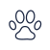• Garage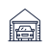•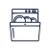•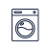•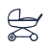• Slopes access :
• ski-in (-50 m)
• Rooms :
• Studio + cabin(s)
• Slopes access :
• 50 -300m to the slopes
• Rooms :
• Studio
• Studio + cabin(s)
• Total surface (sq.m) :
• 23 sq.m
•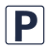••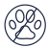• Slopes access :
• 50 -300m to the slopes
• Rooms :
• Studio
• Studio + cabin(s)
• Total surface (sq.m) :
• 19 sq.m
•••• Slopes access :
• ski-in (-50 m)
• Rooms :
• 3 rooms
• Total surface (sq.m) :
• 35 sq.m
••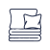•••• Slopes access :
• 50 -300m to the slopes
• Total surface (sq.m) :
• 48 sq.m
•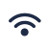•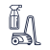•••••• Slopes access :
• 50 -300m to the slopes
• Rooms :
• 3 rooms
• 3 rooms + cabin(s)
• Total surface (sq.m) :
• 67 sq.m
••••• Slopes access :
• ski-in (-50 m)
• Rooms :
• 2 rooms
• Total surface (sq.m) :
• 38 sq.m
•••••• Slopes access :
• 50 -300m to the slopes
• Rooms :
• 2 rooms
• 2 rooms + cabin(s)
• Total surface (sq.m) :
• 48.3 sq.m
••••••• Slopes access :
• ski-in (-50 m)
• Rooms :
• 2 rooms
• Total surface (sq.m) :
• 30 sq.m
••••• Slopes access :
• 50 -300m to the slopes
• Rooms :
• 3 rooms
• Total surface (sq.m) :
• de 60 à 80 sq.m
•••• Slopes access :
• 50 -300m to the slopes
• Rooms :
• 3 rooms
• Total surface (sq.m) :
• 62 sq.m
••• Slopes access :
• 50 -300m to the slopes
• Rooms :
• 3 rooms
• Total surface (sq.m) :
• 80 sq.m
•••••• Slopes access :
• ski-in (-50 m)
• Slopes access :
• ski-in (-50 m)
• Rooms :
• 2 rooms
• Total surface (sq.m) :
• 40 sq.m
•••••• Slopes access :
• ski-in (-50 m)
• Rooms :
• 2 rooms + cabin(s)
• Total surface (sq.m) :
• 32 sq.m
•••• Slopes access :
• ski-in (-50 m)
• Rooms :
• 2 rooms + cabin(s)
• Slopes access :
• ski-in (-50 m)
• Rooms :
• 3 rooms
• Total surface (sq.m) :
• 60 sq.m
••••• Slopes access :
• ski-in (-50 m)
• Rooms :
• 3 rooms
• Total surface (sq.m) :
• 38 sq.m
•••••• Slopes access :
• ski-in (-50 m)
• Rooms :
• 2 rooms + cabin(s)
• Total surface (sq.m) :
• 40 sq.m
••••